# Problem: In this problem, we consider a light clock--a clock that ticks every time light makes a round trip between two mirrors separated by a distance . The key point is that we can deduce the rate at which this clock ticks, when it moves relative to us as well as when it is at rest, directly from the postulates of special relativity. The postulates of special relativity are as follows:The laws of physics are the same in any coordinate system that moves at constant velocity (i.e., any inertial reference frame).The speed of light is  when measured with respect to any coordinate system moving at a constant velocity.As shown in the figure (Intro 1 figure), the light clock is based on the propagation time of light between mirrors spaced a distance  apart (in the rest frame of the clock). As the light bounces back and forth between the mirrors, a small bit of light is allowed to escape through the partially silvered lower mirror. These transmitted pulses of light hit a detector, which therefore emits evenly spaced (in time) "ticks" each round-trip time of the pulse. (New pulses are injected in phase with the detected pulses to keep the clock going.)In this problem, we show that if the clock is moving with speed  relative to a reference frame S, then the ticks from the clock appear slowed when viewed from S. The key is to calculate explicitly the path length of the light pulses as seen in the frame S. From that you can find the length of time that an observer in S will measure between "ticks" of the relatively moving clock.We consider the case where the clock is moving perpendicular to its long axis as shown. Considering a "thought experiment" like this allows us to calculate the tick rate of the clock when viewed by the observer in S. Einstein used thought experiments like this to clarify his thinking as he strove to remove inconsistencies in the basic formulations of physics.Part AWhat is the period  between successive ticks of the clock in its rest frame?Express your answer in terms of variables given in the introduction.We now imagine that the clock is moving with velocity  relative to the observer in S. We know that the pulse of light will travel at speed  in S. Therefore, to calculate the tick rate of the clock, we have to figure out the path of the light in S. The first step is to consider whether the length  appears to change due to relative motion perpendicular to its long axis (i.e., motion perpendicular to ). The situation is shown in the figure, (Intro 2 figure) where the mirror is shown displaced a distance  to where the light pulse hits it, and the source-mirror-detector system is shown where the source emits the pulse and also where it detects the pulse after its first round trip.Part BImagine that pens were mounted beside the mirrors that are  apart in the moving frame. How does the separation of the lines that they would draw on a piece of paper lying on the ground in S compare to , the separation measured in the rest frame of the mirrors?a) It is larger than .b) It is equal to .c) It is less than PART C: Imagine that the mirror separation in S appears some factor  larger than  (i.e., the separation in S is ). Then, by the relativity postulate, the lines drawn on the paper would appear the same factor larger back in the frame of the clock (since the paper appears to move relative to the clock). The clock would then have to have length , which is ridiculous unless  (i.e., the lines are separated by ).We now want to consider the length of the path the light traverses in the clock from the point of view of an observer in reference frameS. One expression for the length of this path is simply: --that is, the length of the path is simply the distance that light travels in one half-tick.Use geometry to find another expression for the length of a one-way trip  (e.g., from the source to the top mirror) according to this observer.Express your answer in terms of the time , , and .Part DWhat is the time  between the ticks of the light clock as viewed from reference frame S?Express the time of these ticks in terms of  (the ticks in the frame of the clock), the relative speed , and the speed of light .

###### FREE Expert Solution

Velocity:

$\overline{){\mathbf{v}}{\mathbf{=}}\frac{\mathbf{∆}\mathbf{x}}{\mathbf{∆}\mathbf{t}}}$

Part A

Δx = 2L

v = c (speed of light)

88% (28 ratings)###### Problem Details

In this problem, we consider a light clock--a clock that ticks every time light makes a round trip between two mirrors separated by a distance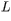. The key point is that we can deduce the rate at which this clock ticks, when it moves relative to us as well as when it is at rest, directly from the postulates of special relativity. The postulates of special relativity are as follows:

1. The laws of physics are the same in any coordinate system that moves at constant velocity (i.e., any inertial reference frame).
2. The speed of light is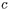when measured with respect to any coordinate system moving at a constant velocity.

As shown in the figure (Intro 1 figure), the light clock is based on the propagation time of light between mirrors spaced a distanceapart (in the rest frame of the clock). As the light bounces back and forth between the mirrors, a small bit of light is allowed to escape through the partially silvered lower mirror. These transmitted pulses of light hit a detector, which therefore emits evenly spaced (in time) "ticks" each round-trip time of the pulse. (New pulses are injected in phase with the detected pulses to keep the clock going.)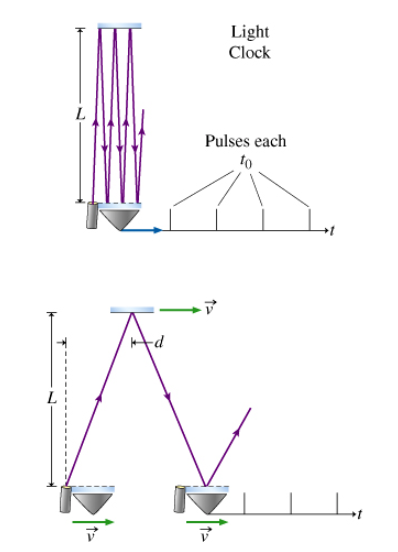In this problem, we show that if the clock is moving with speed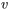relative to a reference frame S, then the ticks from the clock appear slowed when viewed from S. The key is to calculate explicitly the path length of the light pulses as seen in the frame S. From that you can find the length of time that an observer in S will measure between "ticks" of the relatively moving clock.

We consider the case where the clock is moving perpendicular to its long axis as shown. Considering a "thought experiment" like this allows us to calculate the tick rate of the clock when viewed by the observer in S. Einstein used thought experiments like this to clarify his thinking as he strove to remove inconsistencies in the basic formulations of physics.

Part A

What is the period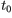between successive ticks of the clock in its rest frame?

We now imagine that the clock is moving with velocityrelative to the observer in S. We know that the pulse of light will travel at speedin S. Therefore, to calculate the tick rate of the clock, we have to figure out the path of the light in S. The first step is to consider whether the lengthappears to change due to relative motion perpendicular to its long axis (i.e., motion perpendicular to). The situation is shown in the figure, (Intro 2 figure) where the mirror is shown displaced a distance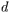to where the light pulse hits it, and the source-mirror-detector system is shown where the source emits the pulse and also where it detects the pulse after its first round trip.

Part B

Imagine that pens were mounted beside the mirrors that areapart in the moving frame. How does the separation of the lines that they would draw on a piece of paper lying on the ground in S compare to, the separation measured in the rest frame of the mirrors?

a) It is larger than.

b) It is equal to.

c) It is less thanPART C: Imagine that the mirror separation in S appears some factor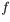larger than(i.e., the separation in S is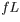). Then, by the relativity postulate, the lines drawn on the paper would appear the same factor larger back in the frame of the clock (since the paper appears to move relative to the clock). The clock would then have to have length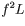, which is ridiculous unless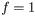(i.e., the lines are separated by).

We now want to consider the length of the path the light traverses in the clock from the point of view of an observer in reference frameS. One expression for the length of this path is simply: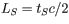--that is, the length of the path is simply the distance that light travels in one half-tick.

Use geometry to find another expression for the length of a one-way trip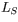(e.g., from the source to the top mirror) according to this observer.

Express your answer in terms of the time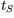,, and.

Part D

What is the timebetween the ticks of the light clock as viewed from reference frame S?

Express the time of these ticks in terms of(the ticks in the frame of the clock), the relative speed, and the speed of light.